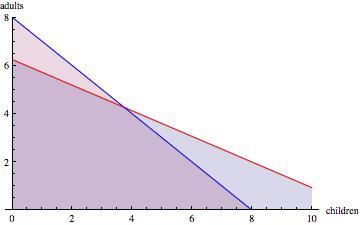# Fishing Adventures 3

Alignments to Content Standards: A-CED.A.3 A-REI.D.12

## Task

Fishing Adventures rents small fishing boats to tourists for day long fishing trips. Each boat can hold at most eight people. Additionally, each boat can only carry 1200 pounds of people and gear for safety reasons. Assume on average an adult weighs 150 pounds and a child weighs 75 pounds. Also assume each group will require 200 pounds of gear plus 10 pounds of gear per person.

1. Write an inequality that illustrates the weight limit for a group of adults and children on the fishing boat and a second inequality that represents the total number of passengers in the fishing boat. Graph the solution set to the inequalities.
2. Several groups of people wish to rent a boat. Group 1 has 4 adults and 2 children. Group 2 has 3 adults and 5 children. Group 3 has 8 adults. Which of the groups, if any, can safely rent a boat? What other combinations of adults and children are possible?

## IM Commentary

This task is the last in a series of three tasks that use inequalities in the same context at increasing complexity in 6th grade, 7th grade and in HS algebra. Students write and solve inequalities, and represent the solutions graphically. The progression of the content standards is 6.EE.8 to 7.EE.4 to A-REI.12.

This particular task could be used for instruction or assessment. This task also has some elements of modeling with mathematics. To find reasonable estimates for the number of people that can rent a boat given certain restrictions, it is necessary to make some simplifying assumptions. In the case of this task we made assumptions about the average weight of adults and children, but this is only one possibility for solving a similar and more open ended problem.

Instead of renting a boat, the problem could also be set in the context of riding an elevator. Being cramped into an elevator often makes you wonder about weight restrictions.

## Solution

1. Let $a=$ number of adults and $c=$ number of children. Then $150a$ is the total weight contributed by the adults, and $75c$ is the total weight contributed by the children. Since 10 pounds of gear is required per adult and per child, we need to add $10a$ and $10c$ to each of these amounts. $$150a+10a=160a$$ $$75c+10c=85c$$ So the total weight in the boat contributed solely by the people is $$160a+85c$$ Because each group also requires 200 pounds of gear regardless of how many people there are, we add 200 to the above amount. We also know that the total weight cannot exceed 1200 pounds. So, we arrive at the following inequality: $$160a+85c+200\leq1200$$ or $$160a+85c\leq1000$$ To write an inequality for the passenger limit in a boat, we observe that the total number of people aboard is the number of adults, $a$, added to the number of children, $c$. Since the number aboard cannot exceed 8, we arrive at $$a+c\leq8$$ We now have a system of linear inequalities: $$160a+85c\leq1000$$ $$a+c\leq8.$$ The graph of the two inequalities is shown below. Note that any solution corresponds to a coordinate point $(c,a)$ that lies in the doubly shaded region and where both coordinates are non-negative integers.2. We can find out which of the groups, if any, can safely rent a boat by first noting that all groups have less than 8 total people, so the passenger limit inequality is satisfied. Substituting the number of adults and children in each group for $a$ and $c$ in our weight inequality, we see that $$\mbox{For Group 1}: 160(4)+85(2)+200=1010\leq1200$$ $$\mbox{For Group 2}: 160(3)+85(5)+200=1105\leq1200$$ $$\mbox{For Group 3}: 160(8)+200=1480\not\leq1200$$ We find that both Group 1 and Group 2 can safely rent a boat, but that Group 3 exceeds the weight limit, and so cannot rent a boat.

We could also have done a visual check to see which of the point $(2,4),\ (5,3),$ and $(0,8)$ lies in the doubly shaded region.

Other combinations of adults and children are possible, and can be found easily by looking at our graph. Any combination where $(c,a)$ lies in the doubly shaded region will work. For example, 6 children and 1 adult or 1 child and 5 adults.